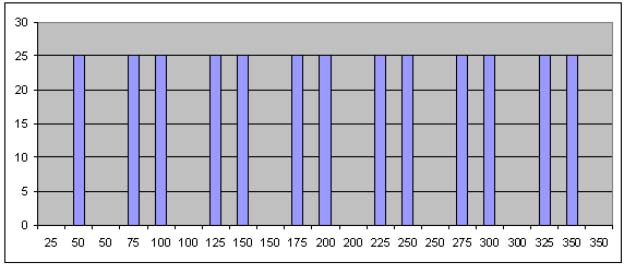»» Resonance and Frequency of a Room

## Resonance and Frequency of a Room

This article discusses: resonance, echo / echo (reverberation), reflectance and position settings. Because of the length of the material covered me divide my article into 4 parts. Which I will start with Resonance in a room.
Resonance is a natural occurrence that is defined as follows: The vibration of an object / mass at a specific frequency due to the sound source. So the air particles in the room heard us resonate at a specific frequency when there is a sound source in the room.

Note: There are a few claims that placing a soundproofing, therefore the problem of resonance will be solved. But this is not true because resonance does not happen due to bounce, but caused due to the vibration of particle that is flanked by the partition.

A. How much resonance frequency in our room?

A listening room generally is made up of 3 surfaces that is parallel which are :

(1) Ceiling and floor,
(2) Front wall and back wall
(3) Left wall and right wall

Resonance frequency that happens on 2 surfaces that parallels can be count with the following formula
Fr = 300 / 2L

Fr = Resonance Frequency
300 = Speed of Sound (meter/second).
L = Distance between the parallel surface.

So if we have a room with the a width of 3 meter therefore the resonance frequency towards the width is as follows:
Fr = (300m/s) / (2 x 3m) = 50Hz

And resonance frequency is followed by the second, third, fourth harmonic frequency and so on.

So If we have a resonance frequency with a 3 meter width room :

Fr1=50Hz; Fr2=100Hz; Fr3=150Hz; Fr4=200Hz; Fr5=250Hz; Fr6=300Hz; Fr7=350Hz.

Where:
Fr2 is the second resonance frequency
Fr3 is the third resonance frequency
? and so on

If the room is a 6 meters therefore we can formulate the formulate the resonance frequency as follows :

Fr = (300m/s) / (2 x 6m) = 25Hz

Fr1=25Hz; Fr2=50Hz; Fr3=75Hz; Fr4=100Hz; Fr5=125Hz; Fr6=150Hz; Fr7=175Hz;
Fr8=200Hz; Fr9=225Hz; Fr10=250Hz; Fr11=300Hz; Fr12=325Hz; Fr13=350Hz

From that we can see that Fr2 resonance frequency room length = 50z and Fr1 frequency width room = 50z is always the same on frequncy 100Hz, 150Hz, 200Hz, 300Hz, 350Hz This causes 50Hz, 100Hz, 150Hz, 200Hz, 250Hz, 300Hz, 350Hz more dominant and thick (Dominant on a certain frequency. On image 1 it can be seen duplication of numbers 50, 100, 150, 200, 250, 300, 350 that is supported in thickening resonance frequency. While on Y axis the number of delta is the same which is 25The resonant frequency response graph hearing room; p = 6m, l = 3m

As a result, natural sound that is generated from the source device that is amplified by an amplifier which has also been natural out through the speakers that is also natural becomes unnatural (response frequency) because the resonance frequency hearing room was very dominant at the frequency of 50Hz, 100 Hz, 150 Hz, 200 Hz, 250 Hz, 300 Hz and so on. Consequently hearing room that we create harmonic distortion during this time we do not realize. This answers the question of why the room with the exact same length and width are not appropriate.

B. How to solve this problem?
How to make a frequency we hear space as natural as possible?

To make the hearing room with the natural frequency / distributed evenly, MM. Louden acoustic experts creates a table

(Table 1). This table contains a comparison between the length and height of the room. Figures x and y for length / width or vice versa.So if we hear the room height is 2.2 meters then: Width space should be 1.4 x 2.2 meter = 3.08 meters and the length should be 1.9 x 2,2meter = 4.18 meters (Table 1 Quality 1 ).

Thus the resonance frequency that occurs is as shown in Table 2 below:If the number of the above table we created in the form of a graph, it would be like the chart below.Resonant frequency response graph at the hearing room; p = 4,18m, 3,08m = l, t = 2,2mDari Figure 2 above shows that the distribution of resonance frequencies that occur fairly evenly in almost all frequencies, and the Y axis (delta) his numbers vary. In this space harmonic distortion that occurs is much smaller than the hearing room on the first discussion.

TIPS: It is very difficult to get a hearing room with the proportion of the length, width and height exactly with tables MM Louden. There are several ways to get the proportions hearing room with an ideal size in accordance with the table MM Louden.

Namely: Creating a platform on the floor so it can be high in ideal space
Creating a partition wall in order to get the length / width ideal
Make the ceiling with a certain slope. Wherein the position of the speakers are on the ceiling lower and higher diplafon listeners.

To see some examples of making slanted ceiling or sloping walls please see www.acourete.com.

And thus the end of the article about resonance frequency. I hope it is useful for many.

### ALTA Integra

Jl. Hayam Wuruk no 2R-S
Jakarta Pusat, 10120
Telp : 021 351 3 351
Fax: 021 345 8 143[/vc_column_text][/vc_column][/vc_row]